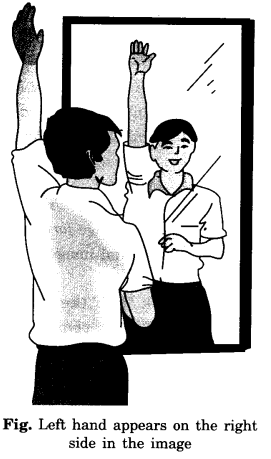NCERT Solutions for Class 7 Science Chapter 15 Light are part of NCERT Solutions for Class 7 Science. Here we have given NCERT Solutions for Class 7 Science Chapter 15 Light.

 Board CBSE Textbook NCERT Class Class 7 Subject Science Chapter Chapter 15 Chapter Name Light Number of Questions Solved 13 Category NCERT Solutions

## NCERT Solutions for Class 7 Science Chapter 15 Light

Question 1.
Fill in the blanks:

1. An image that cannot be obtained on a screen is called ………
2. The image formed by a convex ……… is always virtual and smaller in size.
3. An image formed by a ………. mirror is always of the same size as that of the object.
4. An image which can be obtained on a screen is called a ………. image.
5. An image formed by a concave ………. cannot be obtained on a screen.

1. virtual image
2. mirror
3. plane
4. real
5. lens

Question 2.
Mark “T” if the statement is true and ‘F’ if it is false:

1. We can obtain an enlarged and erect image by a convex mirror. (T/F)
2. A concave lens always forms a virtual image. (T/F)
3. We can obtain a real, enlarged, and inverted image by a concave mirror. (T/F)
4. A real image cannot be obtained on a screen. (T/F)
5. A concave mirror always forms a real image. (T/F)

1. F
2. T
3. T
4. F
5. F

Question 3.
Match the items given in Column I with one or more items of Column II.

 Column I Column II (a) A plane mirror (i) Used as a magnifying glass. (b) A convex mirror (ii) Can form images of objects spread over a large area. (c) A convex lens (iii) Used by dentists to see an enlarged images of teeth. (d) A concave mirror (iv) The image is always inverted and magnified. (e) A concave lens (v) The image is erect and of the same size as the object. (vi) The image is erect and smaller in size than the object.

 Column I Column II (a) A plane mirror (v) The image is erect and of the same size as the object. (b) A convex mirror (ii) Can form images of objects spread over a large area. (c) A convex lens (i) Used as a magnifying glass. (d) A concave mirror (iii) Used by dentists to see enlarged images of teeth. (e) A concave lens (vi) The image is erect and smaller in size than the object.

Question 4.
State the characteristics of the image formed by a plane mirror.
Image formation by a plane mirror. We are able to see images using a mirror. An image formed by a mirror (flat) has the following features:In a plane mirror, the image is formed behind the mirror. It is erect, of the same size, and is at the same distance from the mirror as the object is in front of it.

Question 5.
Find out the letters of the English alphabet or any other language known to you in which the image formed in a plane mirror appears exactly like the letter itself. Discuss your findings.
A, H, I, M, 0, T, U, V, W, X, Y.

Question 6.
What is a virtual image? Give one situation where a virtual image is formed.
The image that cannot be formed on a screen is called a virtual image. The image formed by a plane mirror is virtual because the image cannot be obtained on a screen when placed either in front of the mirror or behind it.

Question 7.
State two differences between a convex and a concave lens.

 Convex lens Concave lens 1. It is thicker in the middle and thinner at the edges. 1. It is thinner in the middle and thicker at the edges. 2. Converges the light falling on it. 2. Diverges the light falling on it. 3. Can form virtual, erect, and magnified images. 3. Always forms erect, virtual, and smaller images.

Question 8.
Give one use each of a concave and a convex mirror.

1. Concave mirror: Used by dentists to see teeth.
2. Convex mirror: Used in vehicles as a rearview mirror.

Question 9.
Which type of mirror can form a real image?
A concave mirror can form a real image.Fig. Convex mirror as a side-view mirror

Question 10.
Which type of lens forms always a virtual image?
A concave lens.

Choose the correct option in questions 11-13:

Question 11.
A virtual image larger than the object can be produced by a
(i) concave lens
(ii) concave mirror
(iii) convex mirror
(iv) plane mirror
(ii) Concave mirror

Question 12.
David is observing his image in a plane mirror. The distance between the mirror and his image is 4 m. If he moves 1 m towards the mirror, then the distance between David and his image will be:
(i) 3 m
(ii) 5 m
(iii) 6 m
(iv) 8 m